# A Brief Overview of Pandas DataFrames

### Get a handle on DataFrames with Pandas and Python.

· Big Data Zone · Tutorial
Save
14.46K ViewsDataframes are going to be the main tool that developers use when working with pandas.

You may also like: Understanding NumPy.

## Prerequisites

Python's pandas module should be installed in the system, and, if it is not already, you can install it using:

``pip install pandas ``

OR

``conda install pandas ``

(if you have Anaconda distribution of python)

## DataFrames and Pandas

DataFrames are the workhorse of pandas and are directly inspired by the R programming language. We can think of a DataFrame as a bunch of Series objects put together to share the same index. Let's use pandas to explore this topic.

``````import pandas as pd
import numpy as np

from numpy.random import randn

np.random.seed(101)``````

To generate some random numbers, we use seed here.

Let’s create a dataframe now:

``df = pd.Dataframe``

If you are using Jupyter Notebook, press shift+tab after  `df = pd.Dataframe` , and you will see this: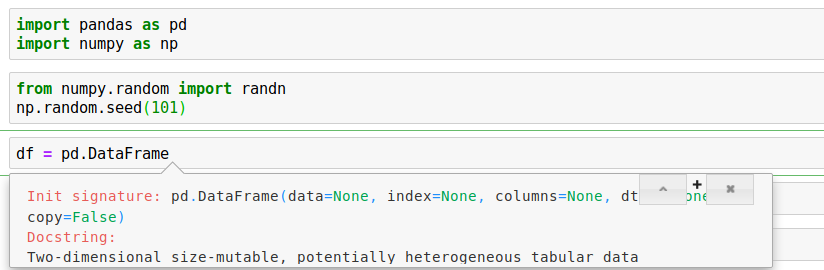Check out the docstring and the initial signature for this DataFrame. We have a data argument, index argument (just like Series), but then we have these additional Columns arguments.

Let's go ahead and create it with some random data, and we'll see what a DataFrame actually looks like. For data, we are using `randn(5,4)` , for index, we are using a list of characters, and for columns, we are using another list of characters.

``df = pd.DataFrame(randn(5,4),['A','B','C','D','E'],['W','X','Y','Z']) ``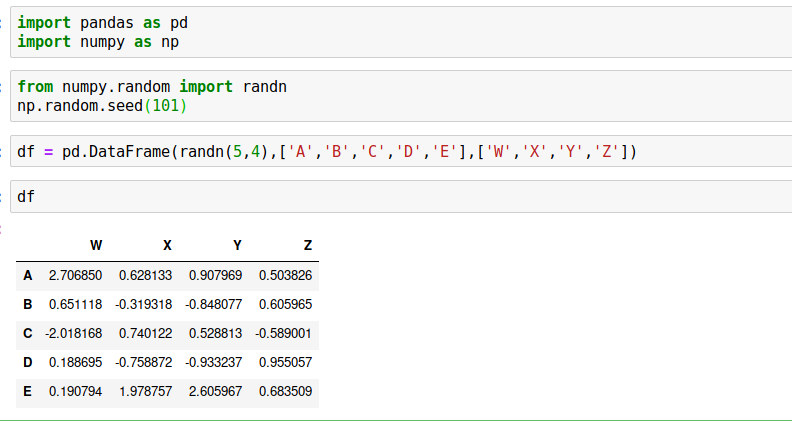So, basically what we have here is a list of columns W, Y, Y, and Z and corresponding rows A, B, C, D, and E. Each of these columns is actually a pandas series, such as W or X or Y or Z, and they all share a common index.

### Selection and Indexing:

Let’s grab data from a DataFrame.

#### Selecting columns:

``df['W']``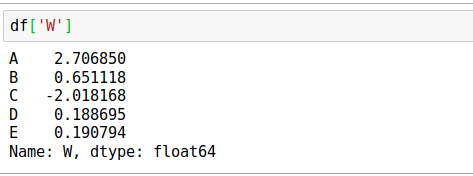You can check the type using:

``type(df['W']) ``

This will give pandas.core.series.Series as a result.

You can also check:

``type(df) ``

which will give pandas.core.frame.DataFrame as a result.

If you want to select multiple columns use:

``df[['W','Z']] ``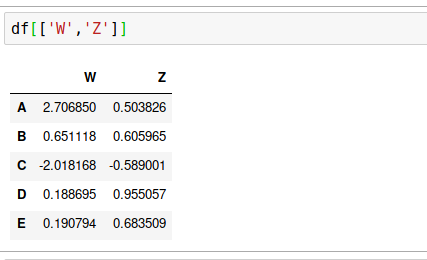### Creating New Columns

``df['new'] = df['W'] + df['Y'] ``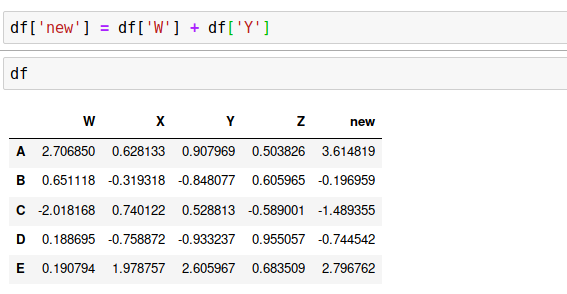### Removing Columns

For removing columns, you can just do:

``df.drop('new',axis=1) ``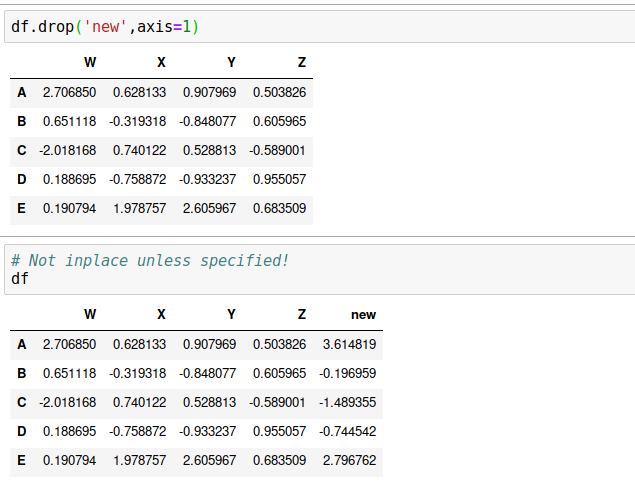Here, you can use shift + tab to check what axis actually refers to. Axis = 0, which is by default is for rows, whereas, Axis = 1 refers to columns. So, here we use axis=1, because we wanted to drop a column.

Note: "new" column still exists; you have to use the `inplace` parameter to retain this change. Pandas does this so users don't accidentally lose information. So, use `inplace = True`.We can also use  `df.drop('E',axis=0)`  to drop a row. Try it yourself.

#### Why are the rows 0 and why are the columns 1?

The reference actually comes back to numpy. DataFrames are essentially index markers on top of a numpy array. Using df.shape() results in a tuple (5, 4). For a two-dimensional matrix, at the 0 index are the number of rows (A,B,C,D,E) and then on the index 1 are columns (W,X,Y,Z). This is why rows are referred to as the 0 axis and columns are referred to as 1 axis because it's directly taken from the shape, just like in a numpy array.

### Selecting Rows

There are two ways to select rows in a DataFrame, and you have to call a method for this.

Select based on label:

`df.loc['A']`

OR

Select based on position:

`df.iloc`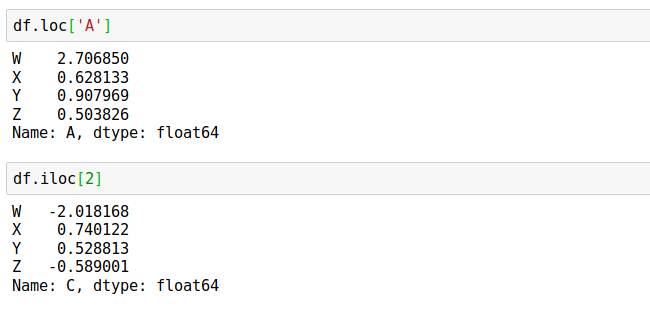Note: Not only are all the columns series but the rows are series as well.

#### Selecting Subsets of Rows and Columns:

For this, you can use:

``df.loc[['A','B'],['W','Y']] ``

For selecting a particular value, use:

`df.loc['B','Y']`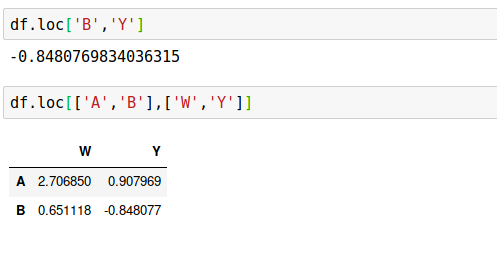### Conditional Selection

A very important feature of pandas is the ability to perform conditional selection using bracket notation. This is going to be very similar to numpy.

Let’s use a comparison operator:

`df > 0`

The result is a DataFrame with boolean values. This returns true if the DataFrame value at that position is greater than zero and false if it is not greater than zero. See below: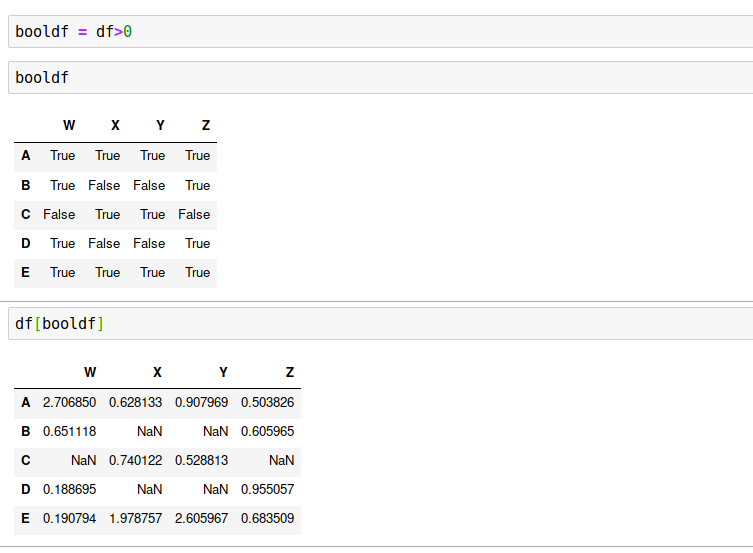`df[df>0]`

As you can see, wherever the value is negative, not satisfying the condition, `NaN` has been returned.

Now, what's important is, instead of returning `NaN`, we will return only the rows or columns of a subset of the DataFrame where the conditions are true.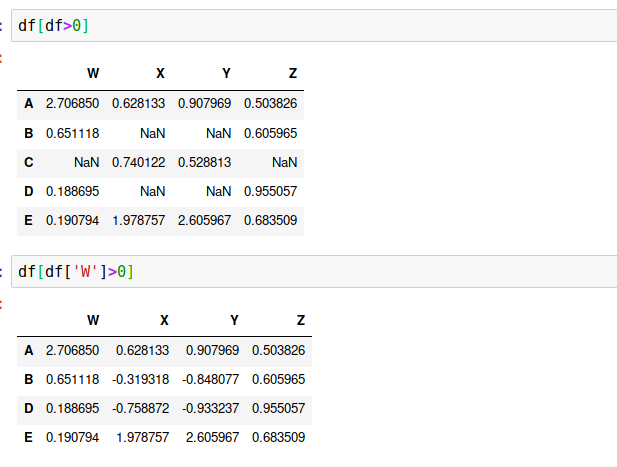Let's say we want to grab information in DataFrame where the column value is

`W>0`

, and we want to extract the "Y" column. We can also select a set of columns such as "Y" and "X," after applying the condition. See below: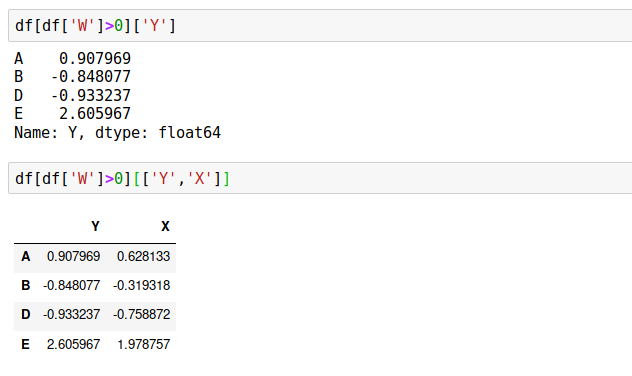#### Using multiple Conditions:

For more than one condition, we can use | or &. Remember that we cannot use python’s and/or here.

``df[(df['W']>0) & (df['Y'] > 1)] ``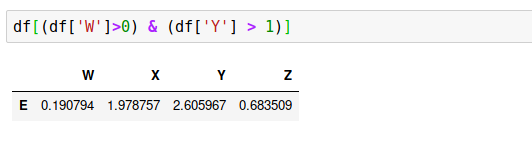### Resetting Indexes

In order to reset the index back to the default which is 1234....n, we use the method reset_index(). We will get the index, reset to a column and the actual index converted to a numerical. But it will not retain the change if you don’t use inplace=True. Pandas uses this inplace argument in many areas, just shift+tab (if using Jupyter Notebook), and you will see it.

``df.reset_index() ``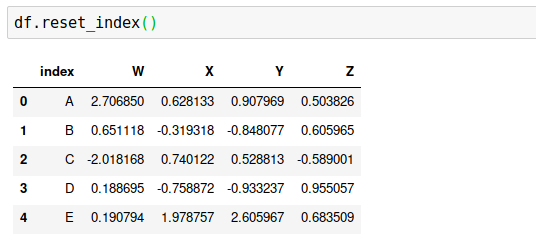### Setting New Index

For setting a new index, first, we have to create a new index. We are using the split() method of a string, which is just a common method for splitting off a blank space. It’s a quick way to create a list ;)

``newind = 'WB MP KA TN UP'.split() ``

Now, put this list as a column of the dataframe.

``````df['States'] = newind
df ``````

If we want to use this State column as the index, we should use:

``df.set_index('States') ``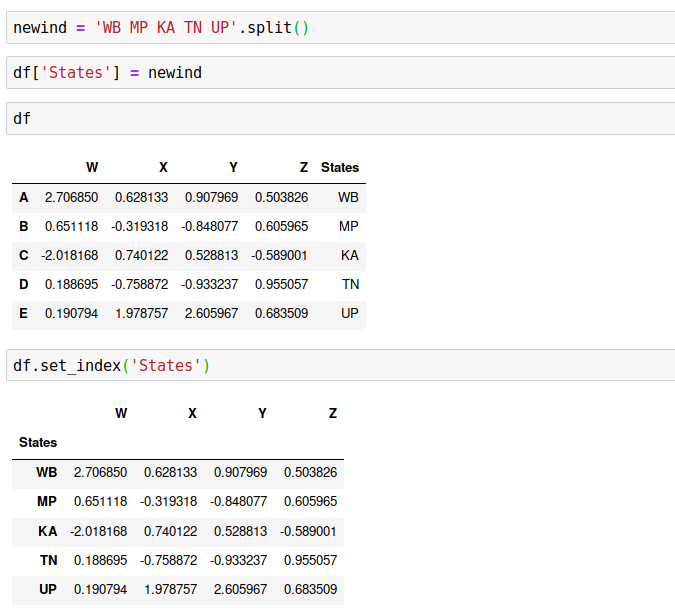Note: Unless we retain this information of the index, it will overwrite the old index, and we won't actually be able to retain this information as a new column — unlike resets index that allows us to have that new column.

So, that's set index versus reset index. :)

Here, inplace=True also plays an important role.

I hope, you have enjoyed reading about DataFrames thus far. There's more to cover in the upcoming article on DataFrames.

Happy learning :)

## Related Articles

Topics:
python 3, pandas data frames tutorial, pandas, pandas tutorial, big data, big data adoption, tutorial, numpy

Opinions expressed by DZone contributors are their own.